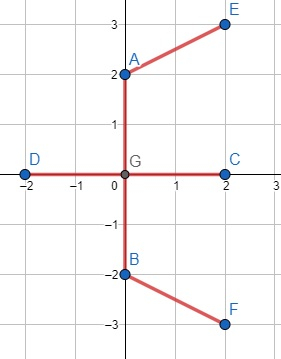# Program to find minimum cost to connect each Cartesian coordinates in C++

Suppose we have a list of 2D Cartesian coordinate points (x, y). We can connect (x0, y0) and (x1, y1), whose cost is |x0 - x1| + |y0 - y1|. If we are allowed to connect any number of points, we have to find the minimum cost necessary such that every point is connected by a path.

So, if the input is like points = [[0, 0],[0, 2],[0, -2],[2, 0],[-2, 0], [2, 3], [2, -3]],then the output will be 14 because, from (0, 0) to (0, 2),(0, -2),(2, 0),(-2, 0), costs are 2, total is 8, and (2, 3) is nearest from (0, 2), cost is |2+1| = 3 and for (2, -3) it is nearest to (0, -2), cost is also 3. so total cost is 8 + 6 = 14.

To solve this, we will follow these steps −

• MAX := inf
• Define a function interval(), this will take i, j, and points array p,
• return |(p[i, x] - p[j, x]) + |p[i, y] - p[j, y]||
• From the main method, do the following −
• n := size of p
• if n < 2, then: return 0
• Define an array called distance with n slots and fill with MAX
• Define an array visited of size n
• distance := 0
• for initialize i := 0, when i < n, update (increase i by 1), do −
• min_d := MAX
• node := 0
• for initialize j := 0, when j < n, update (increase j by 1), do −
• if visited[j] is false and distance[j] < min_d, then −
• min_d := distance[j]
• node := j
• visited[node] := true
• cost := cost + distance[node]
• for initialize j := 0, when j < n, update (increase j by 1), do −
• if visited[j] is false, then −
• d := interval(node, j, p)
• distance[j] := minimum of distance[j] and d
• return cost

## Example

Let us see the following implementation to get better understanding −

#include <iostream>
#include <vector>
#define MAX 99999
using namespace std;

int interval(int i, int j, vector<vector<int>>& p) {
return abs(p[i] - p[j]) + abs(p[i] - p[j]);
}

int solve(vector<vector<int>>& p) {
int n = p.size(), cost = 0;
if (n < 2) return 0;

vector<int> distance(n, MAX);
vector<bool> visited(n);

distance = 0;

for (int i = 0; i < n; i++) {
int min_d = MAX, node = 0;
for (int j = 0; j < n; j++) {
if (!visited[j] && distance[j] < min_d) {
min_d = distance[j];
node = j;
}
}

visited[node] = true;
cost += distance[node];

for (int j = 0; j < n; j++) {
if (!visited[j]) {
int d = interval(node, j, p);
distance[j] = min(distance[j], d);
}
}
}
return cost;
}

int main(){
vector<vector<int>> points = {{0, 0},{0, 2},{0, -2},{2, 0},{-2, 0}, {2, 3}, {2, -3}};
cout << solve(points);
}

## Input

{{0, 0},{0, 2},{0, -2},{2, 0},{-2, 0}, {2, 3}, {2, -3}}

## Output

14